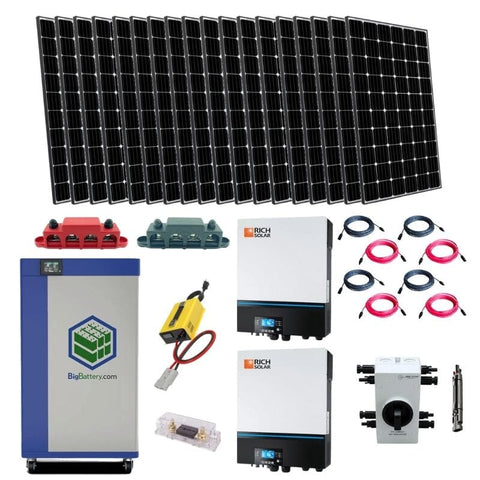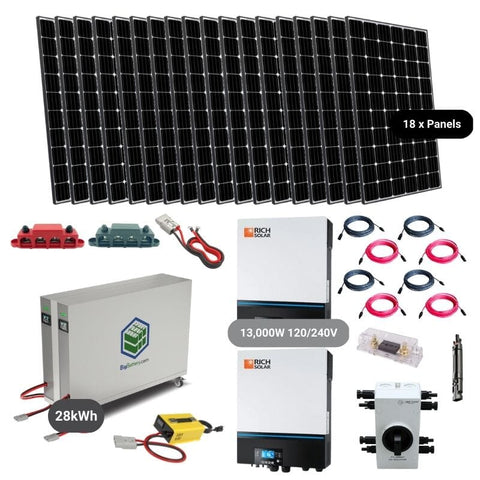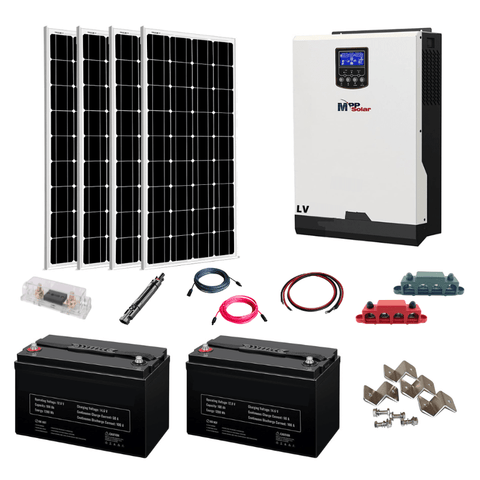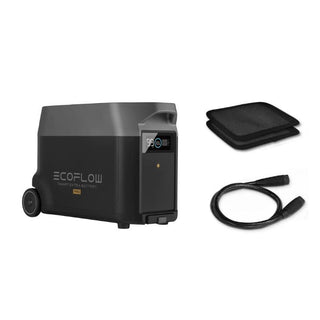Cart# kWh Calculator

By Benjamin Strusnik February 13, 2023

A kWh calculator is a useful way to figure out how much power your home or off-grid solar system consumes. If you want to invest in a solar system to take yourself off of the municipal supply and become truly energy independent, this is where you need to start.

Let’s go over how exactly to do these calculations and all the variables you need.

## Watt Usage Calculator

Before we can look at how to use something like a watts cost calculator, we must understand how to see how many watts an appliance uses.

Watts are derived units of power represented with a capital ‘W’ in calculations. They describe the rate at which work is done on an object.

A kW to amps calculator uses the same electrical law for conversions as a watts calculator: Watt’s Law.

Watt’s Law not only helps us understand how current and voltage share a relationship with wattage but also lets us calculate the watt usage of an appliance.

### Watt’s Law

Watt’s Law states that the power of a circuit is equivalent to the product of voltage and current.

P (Watts) = V (Volts) x I (Amps)

The variables in this equation tend to be shown in the form of a triangle separated into 3 parts.

Power, or watts, is on top, with current and voltage being in the bottom left and right of the triangle respectively.

To calculate the volts, simply covert the voltage section on the triangle and use of the two remaining units. In this case, watts and current.

Since the watts are above the current in the triangle, it’s a matter of division. If they were next to each other then we multiply.

An appliance's power consumption determines the watts an appliance uses for a specific amount of time.

Most appliances have their wattage rated on a power level at the back or bottom of the product, but some only provide you with voltage and amperage.But now that we know what to do if we have volts and amps, the watts can be obtained with ease.

Next, you want to figure out how long you plan on using this appliance.

Now that we’ve got watts out of the way, we can start looking at kW to kWh calculators and kWh usage calculators.

## kWh Usage Calculator

To wrap our heads around exactly what a kWh is, we need to unpack some basic electrical terminology.

Power is defined as work over time, and work is the transfer of energy. Energy is the ability to do work.

Energy is measured in joules whereas power is measured in watts. A single watt is 1 joule per second meaning that energy is power multiplied by time.

Power is also measured in kilowatts, or 1,000 W. Kilowatts multiplied by time give us kilowatt-hours, meaning kWh is a unit of energy.

A kWh usage calculator is like a watt usage calculator but on a larger scale. We use this calculator when we’re working with multiple appliances or entire households rather than a single item.

Let’s see a more practical example.

If we have a laptop that’s using 0.5 kW from our off-grid solar system for 5 hours, the energy usage of this laptop is 2.5 kWh.Knowing the basics about kilowatts and kilowatt hours means we can convert kWh to kW or use other calculators where kW is a variable.

### kWh Per Month Calculator

A kWh per month calculator works like a kWh per square foot calculator. In most cases, your monthly bill shows the consumption of your household in kWh with the cost alongside it.

However, to get a rough estimate of how these values are generated, you can use a kWh per month calculator.

First, make a list of all the appliances you’re running from your solar system. Write down everything from computers to phones and lights.

Once you have the list of appliances, write down their output wattage and convert it to kilowatts. For example, if you have a DC fan in your bathroom and it uses 30 W, it would be 0.03 KW.

Once all your appliances are represented in kW, we can start factoring in time.

You want to write down how many hours per day these appliances are being used.

Multiplying the number of hours by the output kilowattage gives us each appliance's daily kilowatt-hours.

Adding all these values together, we get the total daily kilowatt-hours our household uses.

We want the monthly kilowatt hours so if this value we’ve calculated is a general idea of kWh per day, multiplying that value by 30 gives us the average monthly kWh.## kWh Price Calculator

So we’ve understood the concept of kWh, but unfortunately, nothing comes for free.

If you get your electricity from the grid, you’re going to end up paying the government for the luxury of turning your lights on each month.

The electricity you’re using is distributed locally via a low-voltage network of power lines and transformers. When it reaches your meter, your home is supplied with that electricity to power your devices.

In most states, the general price per kWh is 0.30 cents/kWh. However, in the same way, that gas prices fluctuate every day, electricity and power are no different.

If you want the operating price for a specific appliance, simply obtain the kWh it consumes each month and multiply it by 0.30.

### Watts to kWh Cost Calculator

This calculator is almost identical to the kWh price calculator but you begin by converting watts to kW.

For some people, it’s easier to work using watts and watt-hours and then converting to kWh before calculating the price.The general concept is the same. You determine the watt-hours used per day and multiply it by 30 to get the monthly consumption.

Once we have this value, we can multiply it by 1,000 to get the kWh per month.

From here, multiply it by the cost of electricity in your area and voila: you now know how to use a watts to kWh cost calculator.

## Conclusion

Knowing the ins and outs of a kWh calculator is useful when trying to figure out how long a solar system is going to take to pay itself off.

By using a kWh price calculator, you can see how much money you can save every month by even using a relatively small solar system to power a few of your appliances.

Did You Find Our Blog Helpful? Then Consider Checking:Someone purchased a

#### EcoFlow Delta 1,800W / 1,300wH [Quad Kit] +...

59 minutes agoSomeone purchased a

#### Bluetti AC200P 2,000W / 2,000wH [Hex Kit] +...

59 minutes agoSomeone purchased a

#### Complete Off-Grid Solar Kit - MPP LV2424 2,400W...

59 minutes agoSomeone purchased a

#### Complete Off-Grid Solar Kit - 4,000W 120/240V Output...

59 minutes agoSomeone purchased a

#### Complete Off-Grid Solar Kit - 6,000W 120/240V Output...

59 minutes agoSomeone purchased a

#### EcoFlow DELTA PRO 3,600wH / 3,600W [Hex Kit]...

59 minutes agoSomeone purchased a

#### EcoFlow DELTA PRO [Extra Battery] | 3,600wH Capacity...

59 minutes agoSomeone purchased a

#### EcoFlow DELTA [PRO] 3,600wH / 3,600W [NOMAD Kit]...

59 minutes agoSomeone purchased a

#### EcoFlow DELTA PRO 3,600wH / 3,600W Solar Generator...

59 minutes agoSomeone purchased a

#### Complete DIY Solar Panel Kit - 3,000W Inverter...

59 minutes agoSomeone purchased a

#### Complete Solar Kit - 7,200W 120/240V Output /...

59 minutes agoSomeone purchased a

#### Complete Off-Grid Solar Kit - 6,000W 120/240V Output...

59 minutes agoSomeone purchased a

#### Complete Off-Grid Solar Kit - 6,000W 120/240 Output...

59 minutes agoSomeone purchased a

#### EcoFlow DELTA PRO 3,600wH / 3,600W [Quad Kit]...

59 minutes agoSomeone purchased a

#### Complete DIY Solar Panel Kit - 2,000W Pure...

59 minutes agoSomeone purchased a

#### Complete Hybrid Solar Kit - Sol-Ark 12K 120/240V...

59 minutes agoSomeone purchased a

#### Complete Off Grid Solar Kit - 3,000W /...

59 minutes agoSomeone purchased a

#### Complete Off-Grid Solar Kit - 13,000W 120/240V Output...

59 minutes agoSomeone purchased a

59 minutes ago